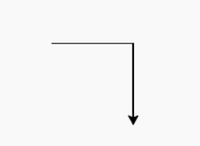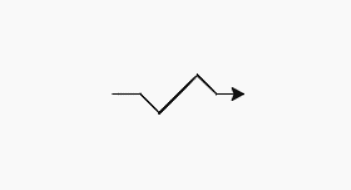Open in App
Not now

# turtle.pos() method in Python

• Last Updated : 01 Aug, 2020

The Turtle module provides turtle graphics primitives, in both object-oriented and procedure-oriented ways. Because it uses Tkinter for the underlying graphics, it needs a version of Python installed with Tk support.

## turtle.pos()

This method is used to find the turtle’s current location (x, y), as a Vec2D-vector. This method has the Aliases: pos | position.

Syntax: turtle.pos() or  turtle.position()

Return: turtle’s current location in terms of (x, y) coordinate

This function does not require any argument and returns the current position of the turtle in the format (x,y) where x and y represent the 2D vector. The default value is (0.0, 0.0).

Below is the implementation of the above method with some examples :

Example 1 :

## Python3

 `# import turtle package``import` `turtle `` ` `# print the default ``# position i.e; (0.0, 0.0)``print``(turtle.pos())`` ` `# forward turtle by 150 pixels``turtle.forward(``150``)`` ` `# print current position ``# i.e; (150.0, 0.0)``print``(turtle.pos())`` ` `# forward turtle by 150 pixels``# after taking turn right``# by 90 degrees``turtle.right(``90``)``turtle.forward(``150``)`` ` `# print position (after next move)``# i.e; (150.0, -150.0)``print``(turtle.pos())`

Output:```(0.0, 0.0)
(150.0, 0.0)
(150.0, -150.0)
```

Example 2:

## Python3

 `# import turtle package``import` `turtle `` ` `# print position (by default)``# i.e; (0.0, 0.0)``print``(turtle.pos())`` ` `# turtle move forward ``# by 40 pixels``turtle.forward(``40``)`` ` `# print position (after move)``# i.e; (150.0, 0.0)``print``(turtle.position())`` ` `# turtle move forward by 40 pixels``# after taking right turn ``# by 45 degrees``turtle.right(``45``)``turtle.forward(``40``)`` ` `# print position``# (after next move) ``print``(turtle.pos())`` ` `# turtle move forward by 80 ``# pixels after taking left``# turn by 90 degrees``turtle.left(``90``)``turtle.forward(``80``)`` ` `# print position``# (after next move) ``print``(turtle.pos())`` ` `# turtle move forward ``# by 40 pixels after taking ``# right turn by 90 degrees``turtle.right(``90``)``turtle.forward(``40``)`` ` `# print position (after next move) ``print``(turtle.position())`` ` `# turtle move forward by ``# 40 pixels after taking ``# left turn by 45 degrees``turtle.left(``45``)``turtle.forward(``40``)`` ` `# print position ``# (after final move) ``print``(turtle.pos())`

Output :```(0.0, 0.0)
(40.0, 0.0)
(68.2842712475, -28.2842712475)
(124.852813742, 28.2842712475)
(153.13708499, 0.0)
(193.13708499, 0.0)
```

My Personal Notes arrow_drop_up